## Tamilnadu Samacheer Kalvi 9th Maths Solutions Chapter 3 Algebra Ex 3.10

9th Maths Exercise 3.10 Solutions Question 1.
Draw the graph for the following
(i) y = 2x
(ii) y = 4x – 1
(iii) y = $$\left(\frac{3}{2}\right)$$ x + 3
(iv) 3x + 2y = 14
Solution:
(i) Put x = -1, y = 2 × -1 = -2
When x = 0, y = 2 × 0 = 0
When x = 1, y = 2 × 1 = 2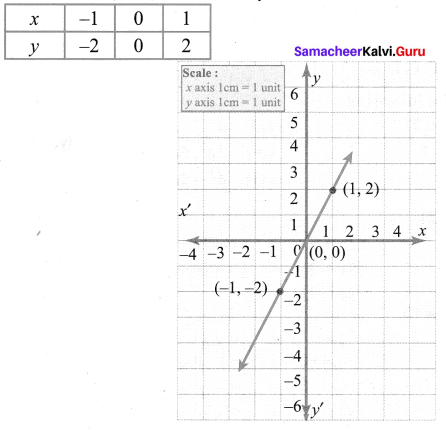The points (x, y) to be plotted: (-1, -2), (0, 0), (1, 2)

(ii) When x = -1 ⇒ y = 4 (-1) -1
y = – 4 – 1 = – 5
x = 0 ⇒ y = 4 × 0 – 1 = -1
x = 1 ⇒ y = 4 × 1 – 1 = 3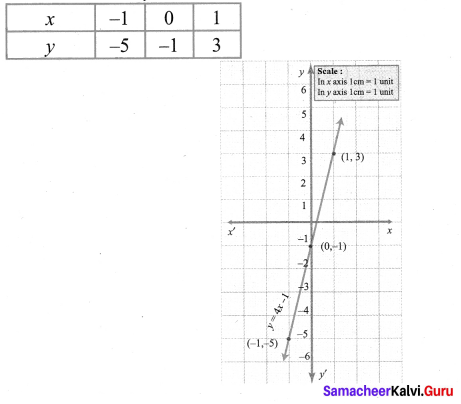The points (x, y) to be plotted: (-1, -5), (0, -1), (1, 3)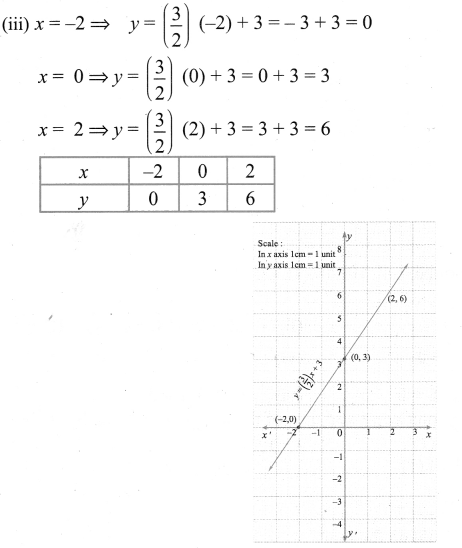The points to be plotted: (-2, 0), (0, 3), (2, 6)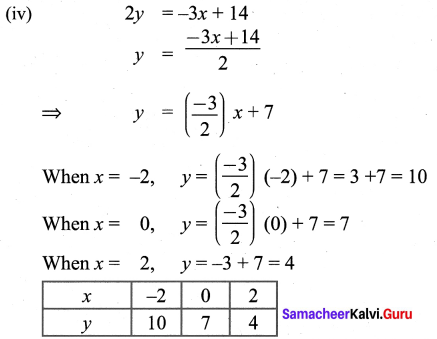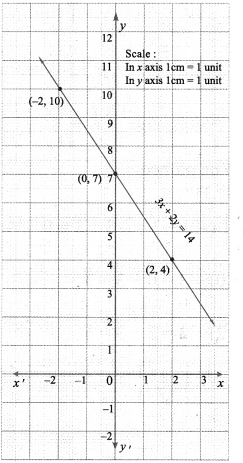The points to be plotted: (-2, 10), (0, 7), (2, 4)

9th Maths Graph Question 2.
Solve graphically
(i) x + y = 7; x – y = 3
(ii) 3x + 2y = 4; 9x + 6y – 12 =0
(iii) $$\frac{x}{2}+\frac{y}{4}=1 ; \frac{x}{2}+\frac{y}{4}=2$$
(iv) x – y = 0; y + 3 = 0
(v) y = 2x + 1; y + 3x – 6 = 0
(vi) x = -3, y = 3
Solution:
(i) We can find x and y intericepts and thus of the two points on the lines (1), (2)
x + y = 7 ……… (1), x – y = 3 …………. (2)
To draw the graph of (1)
Put x = 0 in (1)
0 + y = 7 ⇒ y = 7
Thus A (0, 7) is a point on the line
Put y = 0 in (1)
x + 0 = 7 ⇒ x = 7
Thus B (7, 0) is another point on the line
Plot A and B. Join them to produce the line (1).
To draw the graph of (2), we can adopt the same procedure.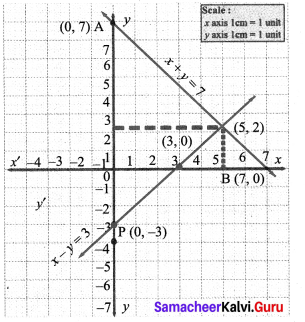When x = 0,(2) ⇒ x – y = 3
0 – y = 3 ⇒ y = -3
P (0, -3) is a point on the line.
Put y = 0 in (2); x – 0 = 3
x = 3
∴ Q (3, 0) is another point on the line (2)
Plot P, Q
1 The point of intersection (5, 2) of lines (1), (2) is a solution

(ii) 3x + 2y = 4 ……. (1)
9x + 6y= 12 ………. (2)
To draw the graph of (1)
Put x = 0 in (1) ⇒ 3 (0) + 2y = 4
2y = 4
y =2
∴ A (0, 2) is a point on the line (1)
Put y = 0 in (1) ⇒ 3x + 2(0) = 4
3x = 4
x = $$\frac{4}{3}$$ = 1.3
∴ B (1.3, 0) is another point on the line (1)
Plot the points A, B. Join them to produce the line (1)
To draw the graph of (2)
Put x = 0 in (2) ⇒ 9 (0) + 6y = 12
6y = 12
y = 2
∴ P (0, 2) is a point on the line (2)
Put y = 0 in (2) ⇒ 9x + 6(0) = 12
9x = 12
x = $$\frac{12}{9}=\frac{4}{3}$$
x = 1.3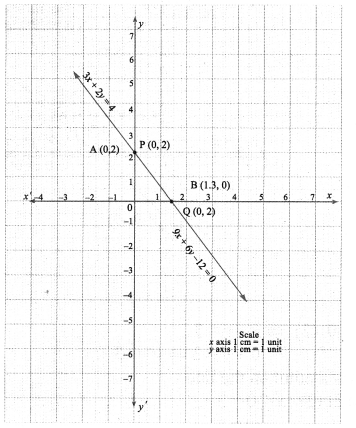Q (1.3, 0) is another point on the line (2)
Plot P, Q. Join them to produce the line (2).
The point of intersection of the lines (1), (2) is a solution.
[A, B],[P, Q] represent the same line.
∴ All the points on one line are also on the other.
This means we have an infinite number of solutions.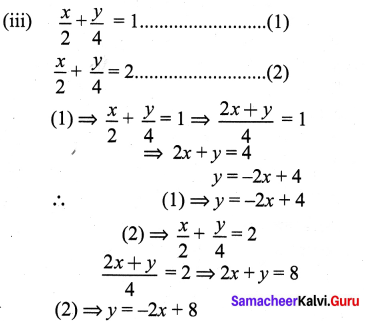∴ Comparing (1), (2) we can conclude their slopes are equal
∴ The lines are parallel and will not meet at any point and hence no solution exists.
Let us draw the graphs of (1) & (2)
(1) ⇒ 2x + y = 4, Put x = 0 in (1) ⇒ y = 4
∴ A (0, 4) is a point on (1)
Put y = 0 in (1) ⇒ 2x = 4
x = 2
∴ B (2, 0) is another point on (1)
Plot A, B ; Join them to produce the line (1)
(2) ⇒ 2x + y = 8, Put x = 0 in (2)
2(0) + y = 8
y = 8, P (0,8) is a point
Put y = 0 in (2) ⇒ 2x + 0 = 8
2x = 8
x = 4, Q (4, 0) is another point on the line (2)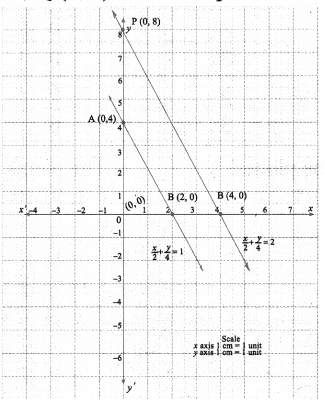Plot P, Q: Join them to produce the line (2)

(iv) x – y = 0 ………….. (1) ⇒ -y = -x ⇒ y = x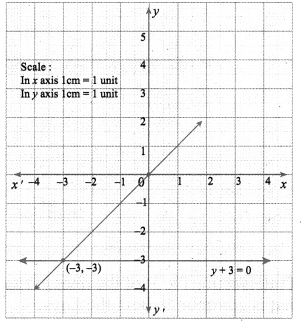Put x = 0 in (1), 0 – y = 0
-y = 0 ⇒ y = 0
A (0, 0) is a point on the line (1)
Put y = 0 in (1) ⇒ x – 0 = 0 ⇒ x = 0
B (-3, -3) is also the same point as A
(2) ⇒ y + 3 = 0
y = -3
∴ from (1) y = -3 = x
∴ B (-3, -3) is the solution

(v) y = 2x + 1 …………… (1)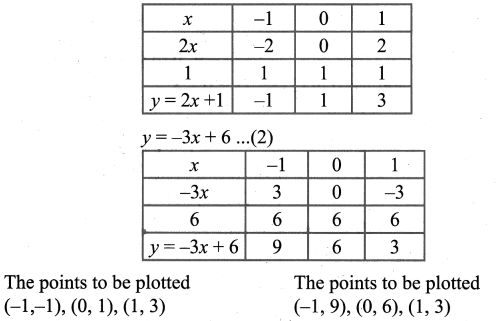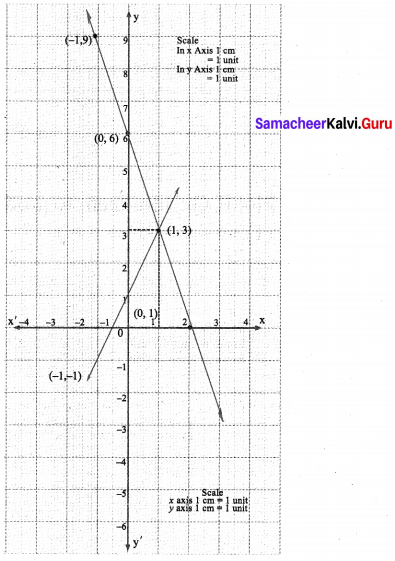The points of intersection (1, 3) of the lines (1) and (2) is a solution. The solution is the point that is common to both the lines.
∴ The solution is as x = 1, y = 3.

(vi) The point of intersection (-3, 3) is a solution.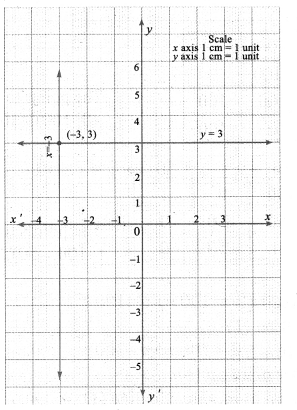x + y = 7 ……… (1), x – y = 3 …………. (2)
To draw the graph of (1)
Put x= 0 in (1)
0 + y = 7 ⇒ y =7
Thus A (0, 7) is a point on the line
Put y = 0 in (1)
x + 0 = 7 ⇒ x =7
Thus B (7, 0) is another point on the line
Plot A and B. Join them to produce the line (1).
To draw the graph of (2), we can adopt the same procedure.
When x = 0, …….. (2) ⇒ x – y = 3
0 – y = 3 ⇒ y = -3
P (0, -3) is a point on the line.
Put y = 0 in (2) ; x – 0 = 3
x = 3
∴ Q (3, 0) is another point on the line (2) Plot P, Q

9th Graph Exercise 3.10 Question 3.
Two cars are 100 miles apart. If they drive towards each other they will meet in 1 hour. If they drive in the same direction they will meet in 2 hours. Find their speed by using graphical method.
Solution:
Let x, y be the speed of the two cars. If the two cars travel towards each other they will meet in 1 hr. The distance between them d = 100; $$\frac{d}{s}$$ = t
i.e., $$\frac{100}{x+y}$$ = 1 ⇒ x + y = 100 ………. (1)
If the two cars travel in the same direction they will meet in 2 hrs.
x + y = $$\frac{100}{2}$$ ⇒ x – y = 50 ………….. (2)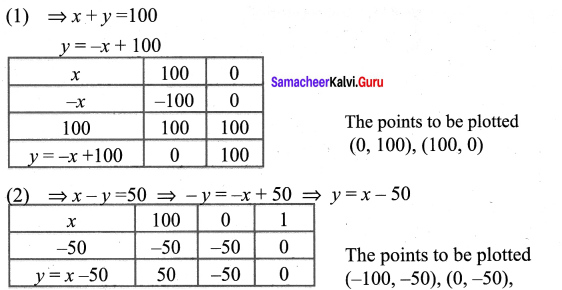x + y = 10 ………….. (1)
Put x = 0 in (1), then 0 + y = 100 ⇒ y =100
A (0, 100) is a point on (1)
Put y = 0 in (1), then x + 0 = 100 ⇒ x =100
B (100, 0) is another point on (1)
Plot A & B. Join them to produce the line (1)
Similarly by x – y = 50
Put x = 0 in (2), then 0 – y = 50 ⇒ y = -50
P (0, -50) is a point on (2)
Put y = 0 in (2), then x – 0 = 50 ⇒ x = 50
Q (50, 0) is another point on (2)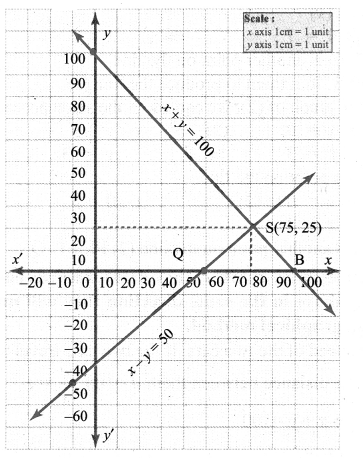Plot P & Q. Join them to produce the line (2)
The point of intersection (75, 25) of the two lines (1) & (2) is the solution.
∴ The solution i.e., the speed of the two cars x and y is given by x = 75 km and y = 25 km